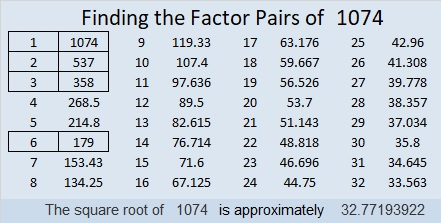# 1074 and Level 2

Jump up and cheer! You can solve this puzzle! Simply write all the numbers from 1 to 10 in both the first column and the top row so that those numbers are the factors of the given clues.Print the puzzles or type the solution in this excel file: 10-factors-1073-1079

Now I’ll tell you something about the number 1074:

• 1074 is a composite number.
• Prime factorization: 1074 = 2 × 3 × 179
• The exponents in the prime factorization are 1, 1, and 1. Adding one to each and multiplying we get (1 + 1)(1 + 1)(1 + 1) = 2 × 2 × 2 = 8. Therefore 1074 has exactly 8 factors.
• Factors of 1074: 1, 2, 3, 6, 179, 358, 537, 1074
• Factor pairs: 1074 = 1 × 1074, 2 × 537, 3 × 358, or 6 × 179
• 1074 has no square factors that allow its square root to be simplified. √1074 ≈ 32.771941074 is the short leg in these Pythagorean triples:
1074-1432-1790 which is (3-4-5) times 358
1074-32032-32050, a primitive calculated from 2(179)(3), 179² – 3², 179² + 3²
1074-96120-96126 which is 6 times (179-16020-16021)
1074-288368-288370 calculated from 2(537)(1), 537² – 1², 537² + 1²

1074 is a palindrome when it is written in two different bases:
It”s 3C3 in BASE 17 (C is 12 base 10) because 3(17²) + 12(17) + 3(1) = 1074,
and 181 in BASE 29 because 29² + 8(29) + 1 = 1074

This site uses Akismet to reduce spam. Learn how your comment data is processed.